# Walsh system

(diff) ← Older revision | Latest revision (diff) | Newer revision → (diff)

of functions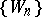on the intervalThe functions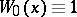andfor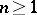, where,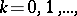are the Rademacher functions (cf. Rademacher system) and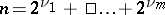,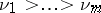, is the binary representation of the number. This system was defined and studied by J.L. Walsh , but already in 1900 J.A. Barrett studied functions of this system in questions connected with the distribution of electrons on open conducting curves. In connection with this theory another definition of Walsh functions is preferred. Namely, if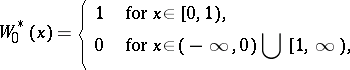then the functions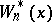are defined by the following recurrence formulas: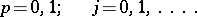The systemsanddiffer only in their ordering in the ranges,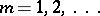. For example,,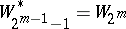,, etc. The indexof the functioncorresponds to the number of changes of sign of this function in the interval, i.e. it is the analogue to doubling the frequency of a sinusoidal function. The Walsh system is a complete orthonormal system on the interval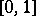and it may be considered as a natural completion of the Rademacher system.

The Walsh system forms a commutative multiplicative group, with the function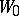as unit element, while each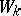is its own inverse.

How to Cite This Entry:
Walsh system. Encyclopedia of Mathematics. URL: http://encyclopediaofmath.org/index.php?title=Walsh_system&oldid=14844
This article was adapted from an original article by A.V. Efimov (originator), which appeared in Encyclopedia of Mathematics - ISBN 1402006098. See original article